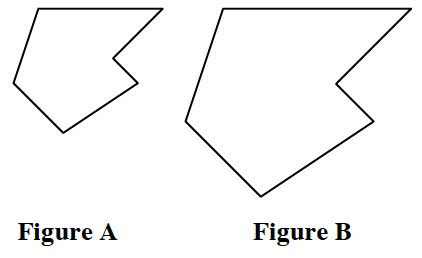### Home > INT2 > Chapter 8 > Lesson 8.3.2 > Problem8-70

8-70.Assume Figure A and Figure B, at right, are similar.

1. If the ratio of similarity is $\frac { 3 } { 4 }$, then what is the ratio of the perimeters of Figures A and B?

$\frac{3}{4}$

2. If the perimeter of Figure A is $p$ and the linear scale factor is $r$, what is the perimeter of Figure B?

If the perimeter of Figure A was $5$ and the scale factor was $3$, how would you find the perimeter of Figure B?

3. If the area of Figure A is $a$ and the linear scale factor is $r$, what is the area of Figure B?

If the linear scale factor is $r$, what is the area ratio?